# High School Chemistry : Phases of Matter

## Example Questions

### Example Question #1 : Using Gay Lussac's Law

If the pressure of a sample of one mole of an ideal gas is increased from 2atm to 3atm at a constant volume, and the initial temperature was 20˚C, what is the final temperature of the sample?

30K

439.5K

195.3K

303K

439.5K

Explanation:

Because the mass and volume of the sample of the ideal gas are kept constant, a change in pressure causes only a direct change in the temperature. This can be derived from the following ideal gas equation: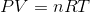.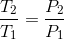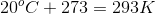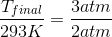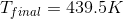### Example Question #1 : Using Gay Lussac's Law

A sample of chlorine gas fills a vessel at a temperature of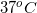. The vessel has a volume of 3L and experiences a pressure of 3atm. What is the mass of the chlorine gas in the vessel?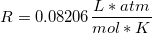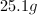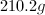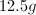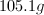Explanation:

This problem requires us to substitute the moles of gas in the ideal gas law to mass over molar mass.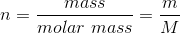We can then isolate for the mass of chlorine gas in the vessel.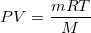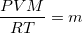Chlorine is diatomic, so its molecular weight will be twice the atomic mass.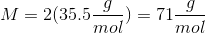The temperature must be expressed in Kelvin.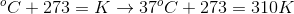Using these values, we can solve for the mass of chlorine gas in the vessel.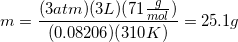### Example Question #1 : Using Gay Lussac's Law

A closed flask contains gas at STP. If the temperature is raised to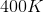what will the pressure be in mmHg?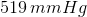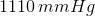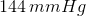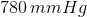Explanation:

Since we have information about temperature and we are interested in changes in pessure, Gay-Lussac's Law will be used. This says that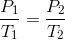The initial temperature and pressure are given (STP) meaning a temperature of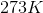and a pressure of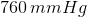. There are other values for standard pressure but they have different units and since the problems requests an answer in units of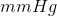we must use the value of standard pressure with those units. Solving for the final pressure gives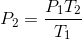Plugging in our variables gives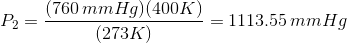We want 3 significant figures since the temperature given has 3, so the final answer is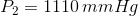### Example Question #1 : Using Avogadro's Law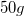of nitrogen gas are contained in a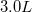container. The gas exerts a pressure of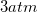on the container.

If pressure is kept constant, what is the final molar amount of gas present in the container if gas is added until the volume has increased to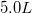?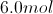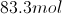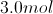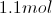Explanation:

Since pressure is kept constant, we can directly compare the moles of gas in the container and volume using Avogadro's law. Since moles of gas and volume are on opposite sides of the ideal gas law, the two variables are directly proportional to one another.

Avogadro's law is written as follows: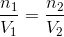First, we will need to convert the initial mass of gas to moles. It is important to remember that nitrogen gas is diatomic!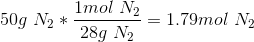Use this value and the given volumes to solve for the final amount of gas in the container.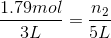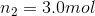### Example Question #2 : Using Avogadro's Law

If 1.0mol of helium gas (He) at standard temperature and pressure (STP) has a volume of 22.4L, how many moles of carbon tetrachloride gas (CCl4) will be present in a container with a volume of 22.4L?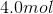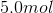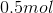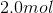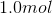Explanation:

Avogadro's Law states that two gases at the same temperature and volume will have an equal number of molecules, and therefore the same number of moles.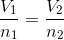It does not matter that helium has one atom per molecule while carbon tetrachloride has five atoms per molecule. Note that the given volume per mole of gas at STP is standard, and true for any gas. At STP, one mole of gas will always have a volume of 22.5L, regardless of its identity.

### Example Question #31 : Gases And Gas Laws

How many neutrons are in 10 moles of aluminum?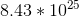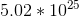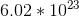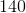Explanation:

To find the number of neutrons, we must convert the moles to atoms by multiplying the moles by Avogadro's number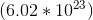.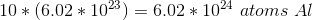Now looking at the periodic table, the neutrons per atom can be found by subtracting the atomic weight by the atomic number: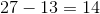Thus there are 14 neutrons in each atom of aluminum.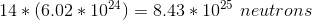### Example Question #1 : Phase Diagrams And Transitions

During the process of freezing, what do you know about changes in the temperature and entropy of water?

The temperature increases and the entropy decreases

The temperature remains constant and the entropy remains constant

The temperature decreases and the entropy decreases

The temperature remains constant and the entropy decreases

The temperature remains constant and the entropy increases

The temperature remains constant and the entropy decreases

Explanation:

The temperature of a sample during the process of a phase change will remain constant. Even though heat may be added or removed during this process, it is utilized to directly impact the formation and breaking of intermolecular interactions within the sample. The result is a change in the internal properties of the sample (the phase), with no actual change in temperature.

When a substance freezes it transitions from a liquid to a solid. The result is a much more ordered structure, as opposed to the fluidity associated with the liquid phase. Increasing order corresponds to a decrease in entropy because entropy is a measure of disorder.

### Example Question #2 : Phase Diagrams And Transitions

Which of the following is associated with freezing, for most substances?

Particles move more quickly and are closer together to each other

Particles move more slowly and are closer to each other

Particles move more slowly and are further apart from each other

Particles move more quickly and are farther apart from each other

Particles move more slowly and are closer to each other

Explanation:

When a substance freezes, the average kinetic energy of the particles decreases. This means that the particles will move more slowly. Most substances also contract when they cool to increase the organization of the molecules (crystallization). Thus, for most substances, particles will get closer together as they freeze. One notable exception is water, which expands as it freezes (the same mass of water that freezes into ice will float in liquid water due to an increase in volume).

### Example Question #3 : Phase Diagrams And Transitions

The following is the phase diagram for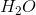.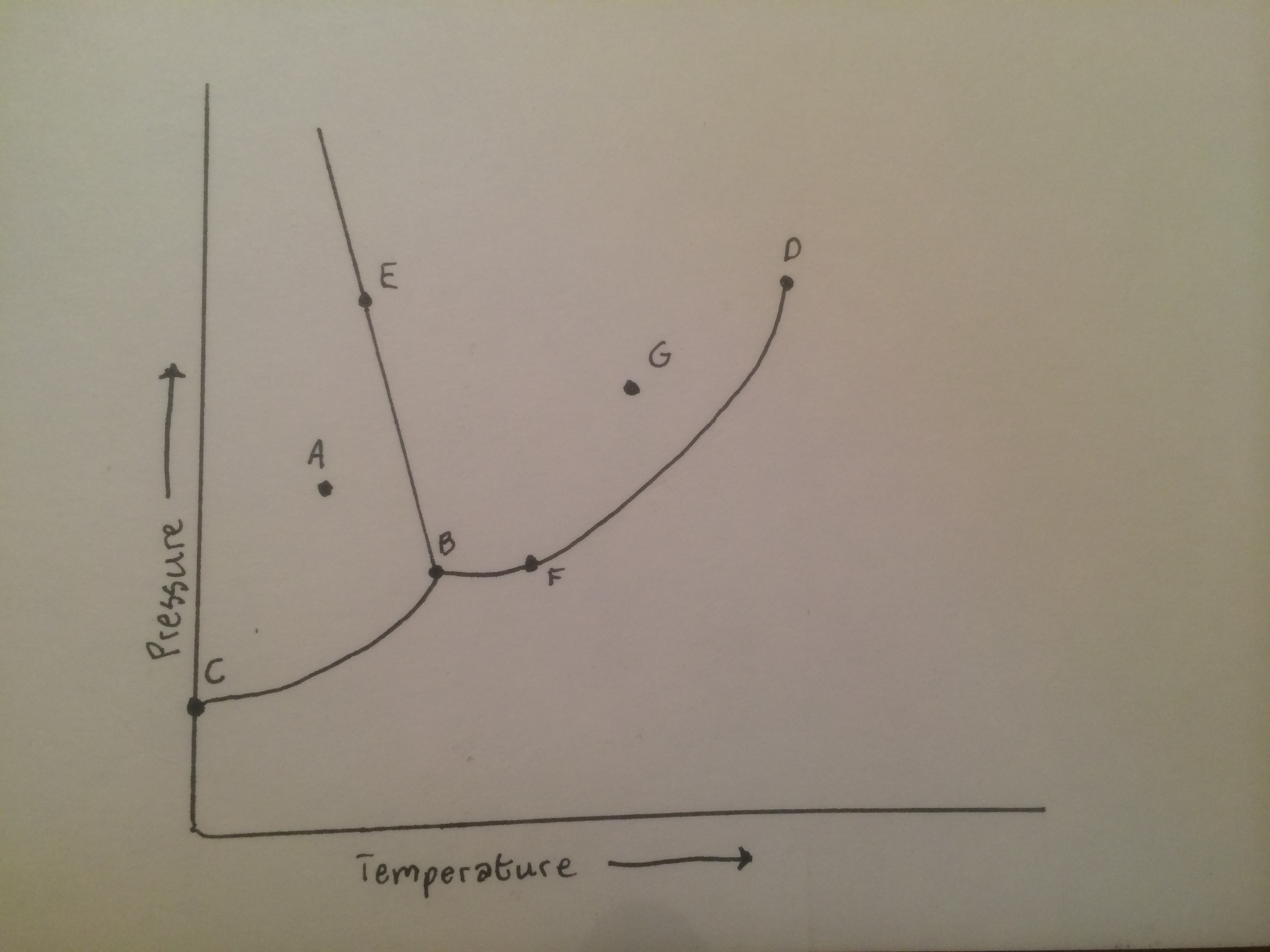What state change is occurring on from point A to point G?

Vaporization

Melting

Condensation

Sublimation

Freezing

Melting

Explanation:

The section point A is in is the solid portion of the phase diagram. At low temperatures and high pressures, substances form solids. Increasing the temperature while keeping pressure high, ice melts into water. Point G is in the liquid portion of the phase diagram.

### Example Question #1 : Help With Condensation And Vaporization

Distillation requires which of the following?

Vaporization only

Either vaporization or condensation

Condensation only

Neither vaporization nor condensation

Vaporization and condensation